﻿ 黄土区裸露坡地径流养分流失模型的建立与验证
 检索项 文章编号 中文标题 英文标题 作者英文名 作者中文名 单位中文名 单位英文名 中文关键词 英文关键词 中文摘要 英文摘要 基金项目 DOI 检索词 1948 1950 1952 1953 1954 1955 1956 1957 1958 1959 1960 1961 1962 1963 1964 1965 1966 1978 1979 1980 1981 1982 1983 1984 1985 1986 1987 1988 1989 1990 1991 1992 1993 1994 1995 1996 1997 1998 1999 2000 2001 2002 2003 2004 2005 2006 2007 2008 2009 2010 2011 2012 2013 2014 2015 2016 2017 2018 到 2018 2017 2016 2015 2014 2013 2012 2011 2010 2009 2008 2007 2006 2005 2004 2003 2002 2001 2000 1999 1998 1997 1996 1995 1994 1993 1992 1991 1990 1989 1988 1987 1986 1985 1984 1983 1982 1981 1980 1979 1978 1966 1965 1964 1963 1962 1961 1960 1959 1958 1957 1956 1955 1954 1953 1952 1950 1948
 土壤学报2021, Vol. 58Issue (4): 900-910  DOI: 10.11766/trxb2020022400690

### 引用本文SHAO Fanfan, WU Junhu, LI Yuchen. Establishment and Validation of the Model for Prediction of Nutrient Loss with Runoff on Bare Slopes in the Loess Plateau. Acta Pedologica Sinica, 2021, 58(4): 900-910.### 作者简介

Establishment and Validation of the Model for Prediction of Nutrient Loss with Runoff on Bare Slopes in the Loess Plateau
SHAO Fanfan, WU Junhu, LI Yuchen
State Key Laboratory of Eco-hydraulics in Northwest Arid Region, Xi'an University of Technology, Xi'an 710048, China
Abstract: 【Objective】Under natural rainfall conditions, a large amount of soil nutrients are losing with overland flow or surface runoff on sloping farmlands in the loess area, which exacerbates the decline of soil quality and productivity of the farmlands and causes serious agricultural non-point source pollution to the environment. Therefore, it is particularly important to accurately predict how soil nutrients loing with surface runoff in the loess areas. However, the existing nutrient loss prediction model focuses on estimating the total nutrient loss over a long period of time in an area, but neglects the effect of infiltrating water diluting the nutrients in the exchange layer before runoff starts.Method In this study, according to the characteristics of nutrient migration in different time periods of a rainfall event, the entire process of a rainfall event is divided into three phases: (1) from the beginning of rainfall (t0) to the time when the exchange layer is completely saturated (tsa), (2) from the time when the exchange layer is completely saturated (tsa) to the time when runoff occurs (tp), and (3) from first runoff (tp) to the end of the rainfall. Based on the approximate analytical solution of the motion wave model, an approximate analytical model of nutrient loss was established, which integrated the effects of raindrop splashing, diffusion and infiltration; and then a simulated rainfall experiment was conducted to determine parameters of the model and verify reliability of the model.Result Results show the values of surface runoff and nutrient loss predicted with the model accurately matched the measured values (R2> 0.8, Nash-Sutcliffe efficiency coefficient> 0.347). In this nutrient loss model, the raindrop-induced water transfer rate er was valued in the range of 0.006~0.023 cm·min-1, and the exchange layer depth de in the range of 0.68~1.32 cm. The former significantly affected peak rate of the nitrate nitrogen and ammonium nitrogen loss, whereas the latter did range of the overall variation of the loss rate and boosted total loss of the nutrients. The model was found to be more sensitive to de than to er.【Conclusion】Some field management measures, such as revegetation, deep fertilization, etc. should be adopted to reduce raindrop kinetic energy and lower the content of nutrients in the exchange layer, so as to realize the purpose of reducing nutrient loss. In general, this approximate semi-analytical model has fully considered the influence of water infiltration in unsaturated soil on nutrient transport in the exchange layer, so it can be used to predict nutrient loss processes on bare slopes in arid and semi-arid regions. However, it should be emphasized that accurate calculation of runoff process is the basis of the nutrient loss simulation, so it is advisable to select an appropriate infiltration formula and solute adsorption coefficients in the light of soil texture, nutrient type, and nutrient concentration in rainwater.
Key words: Model of nutrient loss    Depth of exchange layer    Raindrop splashing    Runoff scouring    Diffusion    Infiltration

1 材料与方法 1.1 理论与模型 1.1.1 坡面径流运动过程

 $\frac{{\partial h}}{{\partial t}} + \frac{{\partial q}}{{\partial x}} = p - i$ (1)

 $q(x, t)=(1-c)\left(p-\frac{1}{2} S(t-\Delta t)^{-1 / 2}\right) x$ (2)

 $h(x, t)=\left(\frac{1}{n} S_{0}^{1 / 2}\right)^{-3 / 5}\left\{(1-c)\left(p-\frac{1}{2} S(t-\Delta t)^{-1 / 2}\right) x\right\}^{3 / 5}$ (3)

1.1.2 径流养分流失过程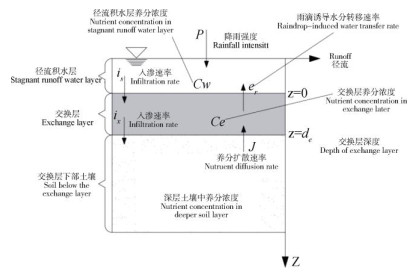图 1 模型物理和化学传输过程的简单示意 Fig. 1 Sketch of physical and chemical transport processes of the model

 $\frac{{d(\alpha {d_e}{C_e})}}{{dt}} = J + {e_r}(\lambda {C_w} - {C_e}) + {i_s}{C_w} - {i_x}{C_e}$ (4)

 $J = - ( - {D_s}\frac{{\partial {C_s}}}{{\partial z}})$ (5)
 ${D_s}\frac{{\partial {C_s}}}{{\partial z}}\left| {_{x = 0} = {C_0}\exp ( - \beta t)(\frac{{\beta \sqrt \alpha }}{{\sqrt {\pi {D_s}} }})} \right.\int_0^t {\frac{\beta }{{\sqrt y }}} dy$ (6)
 $\alpha = \gamma K + {\theta _s}$ (7)

 ${t_{sa}} = \frac{{{d_e}({\theta _s} - {\theta _0})}}{p}$ (8)

 $\begin{array}{l} {C_e}(t) = {C_0}\exp \left( { - A(t - {t_{sa}})} \right) + \frac{{{A^2}}}{{{i_x}}}\sqrt {\frac{{\alpha {D_s}}}{\pi }} \exp \\ \left( { - A(t - {t_{sa}})} \right)\int_{{t_{sa}}}^t {\exp \left( {A(y - {t_{sa}})} \right)\left( {\frac{{t - {t_{sa}}}}{{\sqrt {y - {t_{sa}}} }} - \sqrt {y - {t_{sa}}} } \right)} dy \\ \end{array}$ (9)

 $\begin{array}{l} {C_e}({t_p}) = {C_0}\exp \left( { - A({t_p} - {t_{sa}})} \right) + \frac{{{A^2}}}{{{i_x}}}\sqrt {\frac{{\alpha {D_s}}}{\pi }} \exp \\ \left( { - A({t_p} - {t_{sa}})} \right)\int_{{t_{sa}}}^{{t_p}} {\exp \left( {A(y - {t_{sa}})} \right)\left( {\frac{{{t_p} - {t_{sa}}}}{{\sqrt {y - {t_{sa}}} }} - \sqrt {y - {t_{sa}}} } \right)} dy \\ \end{array}$ (10)

 $\begin{array}{l} {C_e}(t) = {C_e}({t_p})\exp \left( { - B(t - {t_p})} \right) + \frac{{{B^2}}}{{{e_r}}}\sqrt {\frac{{\alpha {D_s}}}{\pi }} \exp \\ \left( { - B(t - {t_p})} \right)\int_{{t_p}}^t {\exp \left( {B(y - {t_p}} \right)\left( {\frac{{t - {t_p}}}{{\sqrt {y - {t_p}} }} - \sqrt {y - {t_p}} } \right)} dy \\ \end{array}$ (11)

 $\frac{{\partial (h{C_w})}}{{\partial t}} + \frac{{\partial q{C_w}}}{{\partial x}} = {e_r}({C_e} - \lambda {C_w}) - {i_s}{C_w}$ (12)

 $h\frac{{\partial {C_w}}}{{\partial t}} + q\frac{{\partial {C_w}}}{{\partial x}} = {e_r}({C_e} - \lambda {C_w}) - p{C_w}$ (13)

 $h\frac{{\partial {C_w}}}{{\partial t}} = {e_r}{C_e} - p{C_w}$ (14)

 $\begin{array}{l} {C_w}(t) = \exp \left( {\int_{{t_p}}^t { - \frac{p}{{h(t)}}dt} } \right) \\ \left( {{C_e}({t_p}) + \int_{{t_p}}^t {\frac{{{e_r}{C_e}(t)}}{{h(t)}}\exp \left( {\int_{{t_p}}^t {\frac{p}{{h(t)}}dt} } \right)} dt} \right) \\ \end{array}$ (15)

 ${M_w}(t) = q(x, t){C_w}(t)x$ (16)

1.2 试验区概况表 1 试验区土壤物理化学特性 Table 1 Physical and chemical properties of the soil tested
1.3 试验方法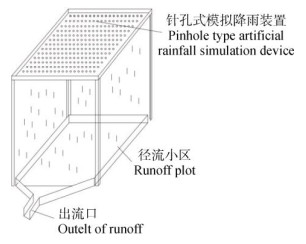图 2 针孔式模拟降雨装置 Fig. 2 Pinhole type artificial rainfall simulation device表 2 模型基本参数 Table 2 Basic parameters of the calculation model
1.4 模型基本参数

1.5 数据处理

2 结果与讨论 2.1 产流过程分析及模拟 2.1.1 产流过程分析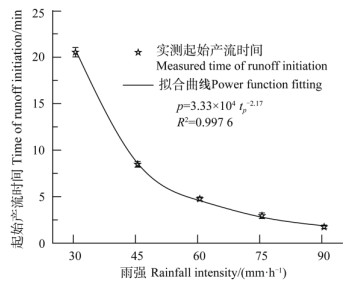注：p是降雨强度；tp是起始产流时间。Note：p is rainfall intensity；tp is the time of runoff initiation. 图 3 不同降雨强度下的产流时间 Fig. 3 Time of runoff initiation relative to rainfall intensity. Bars are means ± standard deviation.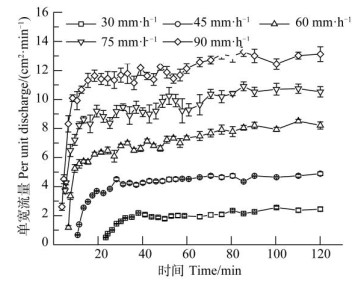图 4 实测单宽流量变化过程 Fig. 4 Variation of measured per unit discharge. Bars are means ± standard deviation.
2.1.2 产流过程模拟表 3 入渗率参数c的最佳拟合值 Table 3 Optimal fitting values of c, R2, RMSE and NSE relative to rainfall intensity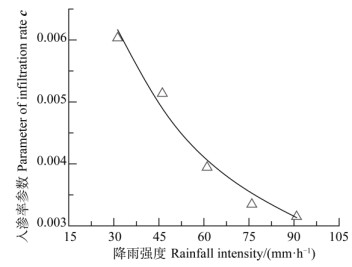图 5 降雨强度与入渗率参数c的关系 Fig. 5 Relationship between rainfall intensity and parameter of infiltration rate, c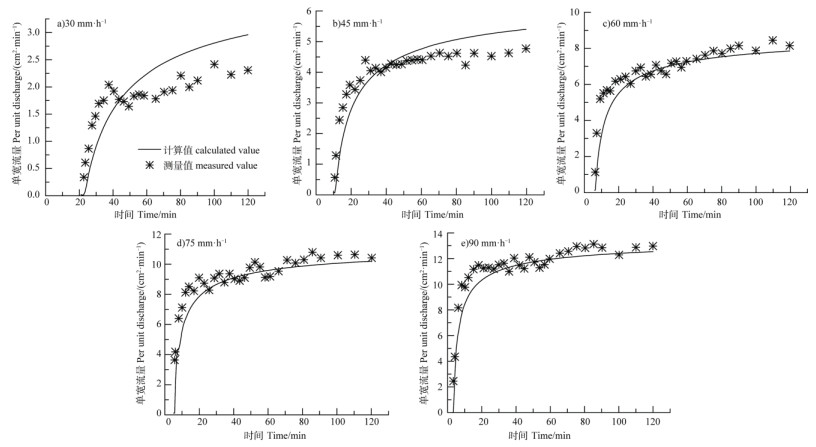图 6 模拟不同降雨强度下的单宽流量变化过程 Fig. 6 Simulated variation of per unit discharge relative to rainfall intensity
2.2 养分流失过程分析及模拟 2.2.1 养分随径流流失过程分析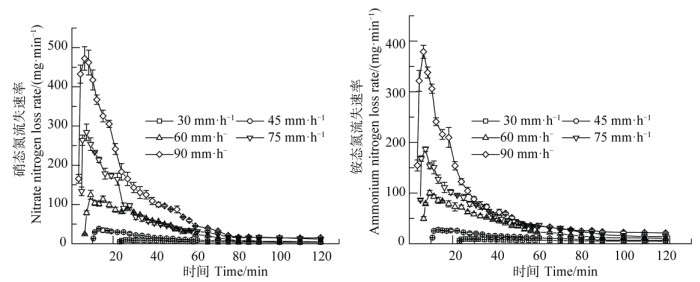图 7 不同降雨强度下硝态氮和铵态氮流失过程 Fig. 7 Processes of nitrate and ammonium nitrogen losses relative to rainfall intensity. Bars are means ± standard deviation
2.2.2 养分流失过程模拟表 4 不同降雨强度下参数deer的最佳拟合值 Table 4 Optimal fitting values of de, er, R2, RMSE and NSE relative to rainfall intensity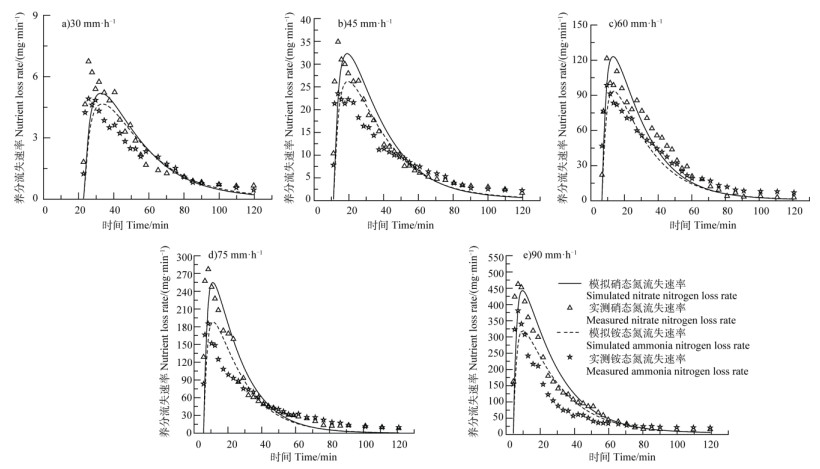图 8 模拟不同降雨强度下的养分流失速率变化过程 Fig. 8 Simulated variation of nutrient loss rate relative to rainfall intensity

3 结论

  Ni S M, Zhang D Q, Feng S Y, et al. Quantitative relationship between hydraulics parameters and soil erosion rate on remolded soil slopes with different textures (In Chinese)[J]. Acta Pedologica Sinica, 2019, 56(6): 1336-1346. [倪世民, 张德谦, 冯舒悦, 等. 不同质地重塑土坡面水沙定量关系研究[J]. 土壤学报, 2019, 56(6): 1336-1346.] (0)  Yang B, Wang W L, Guo M M, et al. Study on runoff and sediment reducing effects of erosion control measures on slopes of slag dumps with simulated rainfall (In Chinese)[J]. Acta Pedologica Sinica, 2017, 54(6): 1357-1368. [杨波, 王文龙, 郭明明, 等. 模拟降雨条件下弃渣体边坡不同防护措施的减水减沙效益[J]. 土壤学报, 2017, 54(6): 1357-1368.] (0)  Shao F F, Wu J H. Earthworm casts on sediment yield and nitrate nitrogen loss with the runoff in slope farmland of loess area (In Chinese)[J]. Journal of Soil and Water Conservation, 2020, 34(1): 71-77. [邵凡凡, 吴军虎. 蚯蚓粪对黄土区坡耕地径流产沙和硝态氮流失特征的影响[J]. 水土保持学报, 2020, 34(1): 71-77.] (0)  Ahuja L R, Sharpley A N, Yamamoto M, et al. The depth of rainfall-runoff-soil interaction as determined by 32P[J]. Water Resources Research, 1981, 17(4): 969-974. DOI:10.1029/WR017i004p00969 (0)  Ahuja L R, Lehman O R. The extent and nature of rainfall-soil interaction in the release of soluble chemicals to runoff[J]. Journal of Environmental Quality, 1983, 12(1): 34-40. (0)  Gao B, Todd Walter M, Steenhuis T S, et al. Investigating ponding depth and soil detachability for a mechanistic erosion model using a simple[J]. Journal of Hydrology, 2003, 277(1/2): 116-124. (0)  Wang Q J, Shao M A, Li Z B, et al. Analysis of simulating methods for soil solute transport with runoff in loess plateau (In Chinese)[J]. Research of Soil and Water Conservation, 1999, 6(2): 67-71, 104. [王全九, 邵明安, 李占斌, 等. 黄土区农田溶质径流过程模拟方法分析[J]. 水土保持研究, 1999, 6(2): 67-71, 104.] (0)  Dong W C, Wang Q J, Zhou B B, et al. A simple model for the transport of soil-Dissolved chemicals in runoff by raindrops[J]. Catena, 2013, 101: 129-135. DOI:10.1016/j.catena.2012.10.007 (0)  Yang T, Wang Q J, Wu L S, et al. A mathematical model for the transfer of soil solutes to runoff under water scouring[J]. Science of the Total Environment, 2016, 569/570: 332-341. DOI:10.1016/j.scitotenv.2016.06.094 (0)  Tong J X, Yang J Z, Hu B X, et al. Experimental study and mathematical modelling of soluble chemical transfer from unsaturated/saturated soil to surface runoff[J]. Hydrological Processes, 2010, 24(21): 3065-3073. DOI:10.1002/hyp.7722 (0)  Tong J X, Hu B X, Yang J Z. Using an ensemble Kalman filter method to calibrate parameters and update soluble chemical transfer from soil to surface runoff[J]. Transport in Porous Media, 2012, 91(1): 133-152. DOI:10.1007/s11242-011-9837-3 (0)  An M Y, Han Y G, Xu L, et al. KINEROS2-based simulation of total nitrogen loss on slopes under rainfall events[J]. Catena, 2019, 177: 13-21. DOI:10.1016/j.catena.2019.01.039 (0)  Ao C, Yang P L, Zeng W Z, et al. Impact of raindrop diameter and polyacrylamide application on runoff, soil and nitrogen loss via raindrop splashing[J]. Geoderma, 2019, 353: 372-381. DOI:10.1016/j.geoderma.2019.07.026 (0)  Luce C H, Cundy T W. Modification of the kinematic wave-Philip infiltration overland flow model[J]. Water Resources Research, 1992, 28(4): 1179-1186. DOI:10.1029/91WR03131 (0)  Yang T, Wang Q J, Su L J, et al. An approximately semi-analytical model for describing surface runoff of rainwater over sloped land[J]. Water Resources Management, 2016, 30(11): 3935-3948. DOI:10.1007/s11269-016-1400-0 (0)  Gao B, Todd Walter M, Steenhuis T S, et al. Rainfall induced chemical transport from soil to runoff: Theory and experiments[J]. Journal of Hydrology, 2004, 295(1/2/3/4): 291-304. (0)  Morooka Y, Cheng D W, Yoshimi K, et al. Proposal and application of a new theoretical framework of uncertainty estimation in rainfall runoff process based on the theory of stochastic process[J]. Procedia Engineering, 2016, 154: 589-594. DOI:10.1016/j.proeng.2016.07.556 (0)  Li J Y, Tong J X, Xia C, et al. Numerical simulation and experimental study on farmland nitrogen loss to surface runoff in a raindrop driven process[J]. Journal of Hydrology, 2017, 549: 754-768. DOI:10.1016/j.jhydrol.2017.01.035 (0)  Wu J H, Shao F F, Liu X. Effects of earthworm casts on soil aggregate composition and water transport during infiltration (In Chinese)[J]. Journal of Soil and Water Conservation, 2019, 33(3): 81-87. [吴军虎, 邵凡凡, 刘侠. 蚯蚓粪对土壤团聚体组成和入渗过程水分运移的影响[J]. 水土保持学报, 2019, 33(3): 81-87.] (0)  Tao W H, Wu J H. Study on numerical simulation of slope runoff and sediment yield rule (In Chinese)[J]. Journal of Soil and Water Conservation, 2016, 30(1): 54-57. [陶汪海, 吴军虎. 坡地产流产沙规律数值模拟研究[J]. 水土保持学报, 2016, 30(1): 54-57.] (0)  Tao W H, Wang Q J, Lin H. An approximate analytical solution for describing surface runoff and sediment transport over hillslope[J]. Journal of Hydrology, 2018, 558: 496-508. DOI:10.1016/j.jhydrol.2018.01.054 (0)  Tao W H, Wu J H, Wang Q J. Mathematical model of sediment and solute transport along slope land in different rainfall pattern conditions[J]. Scientific Reports, 2017, 7: 44082. DOI:10.1038/srep44082 (0)  Yang T, Wang Q J, Wu L S, et al. A mathematical model for soil solute transfer into surface runoff as influenced by rainfall detachment[J]. Science of the Total Environment, 2016, 557/558: 590-600. DOI:10.1016/j.scitotenv.2016.03.087 (0)  Jiang Y F, Ye Y C, Guo X, et al. Spatial distribution of soil available phosphorus and its driving factors under different erosion degrees in South China (In Chinese)[J]. Soils, 2018, 50(5): 1013-1021. [江叶枫, 叶英聪, 郭熙, 等. 南方红壤区不同侵蚀程度下耕地土壤有效磷空间分布特征及其驱动因素[J]. 土壤, 2018, 50(5): 1013-1021.] (0)  Mao N, Huang L M, Shao M A. Profile distribution of soil saturated hydraulic conductivity and controlling factors under different vegetations on slope in loess region (In Chinese)[J]. Soils, 2019, 51(2): 381-389. [毛娜, 黄来明, 邵明安. 黄土区坡面尺度不同植被类型土壤饱和导水率剖面分布及影响因素[J]. 土壤, 2019, 51(2): 381-389.] (0)  Shao F F, Wu J H. Approximate semi-analytical model for surface runoff and sediment yield of idle sloping farmland in Loess Area (In Chinese)[J]. Journal of Soil and Water Conservation, 2020, 34(3): 120-128. [邵凡凡, 吴军虎. 黄土区空闲坡耕地地表径流产沙近似解析模型[J]. 水土保持学报, 2020, 34(3): 120-128.] (0)